• Send Message

Buddies for Life in the Virtual World

View All Friends

••••••••••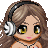Forever Asian (AsianGem)Time to say "WAZZZZUP"

Report | 06/14/2009 12:15 pm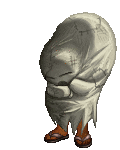hey Joanna
Report | 05/25/2009 5:52 pmOMG SIS U LOOOK HOT AS AVL.
Report | 05/03/2009 1:28 pmhey try this out! send this comment to ten profiles then press f5 to get 10,000 gold
Report | 05/03/2009 1:17 pmhey try this out! send this comment to ten profiles then press f5 to get 10,000 gold
Report | 04/29/2009 12:50 pmhi
Report | 04/26/2009 10:09 amsup!
Report | 04/26/2009 9:17 am:::::::::::::::::::::::::::::::::::::::::::::::::::::::::::::::::::::::::::::::::::::::::::::::::::::::::::::::::::::::::::::::::::::::::::::::::::::::::::::::::::::::::::::::::::::::::::::::::::::::::::::::::::::::::::::::::::::::::::::::::::::::::::::::::::::::::::::::::::::::::::::::::::::::::::::::::::::::::::::::::::::::::::::::::::::::::::::::::::::::::::::::::::::::::::::::::::::::::::::::::::::::::::::::::::::::::::::::::::::::::::::::::::::::::::::::::::::::::::::::::::::::::::::::::::::::::::::::::::::::::::::::::::::::::::::::::::::::::::::::::::::::::::::::::::::::::::::::::::::::::::::::::::::::::::::::::::::::::::::::::::::::::::::::::::::::::::::::::::::::::::::::::::::::::::::::::::::::::::::::::::::::::::::::::::::::::::::::::::::: smile ))))))))))))))))))))))))))))))))))))))))))))))))))))))))))))))))))))))))))))))))))))))))))))))))))))))))))))))))))))))))))))))))))))))))))))))))))))))))))))))))))))))))))))))))))))))))))))))))))))))))))))))))))))))))))))))))))))))))))))))))))))))))))))))))))))))))))))))))))))))))))))))))))))))))))))))))))))))))))))))))))))))))))))))))))))))))))))))))))))))))))))))))))))))))))))))))))))))))))))))))))))))))))))))))))))))))))))))))))))))))))))))))))))))))))))))))))))))))))))))))))))))))))))))))))))))))))))))))))))))))))))))))))))))))))))))))))))))))))))))))))))))))))))))))))))))))))))))))))))))))))))))))))))))))))))))))))))))))))))))))))))))))))))))))))))))))))))))))))))))))))))))))))))))))))))))))))))))))))))))))))))))))))))))))))))))))))))))))))))))
Report | 04/26/2009 9:12 amkk
Report | 04/26/2009 9:10 amu dont have to
smile
Report | 04/26/2009 9:02 amu could have told me i ben sitin her like a dumd azzz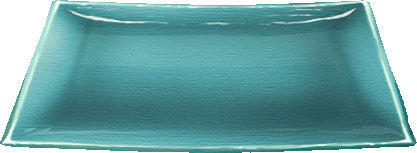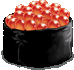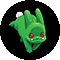Ang Gandana Philipinas! I Love Asians!

It's dark outside
Not nearly as bright
As the sunsets in California
It's colder than night
Nothing here feels right
And nearby the clouds are
Rolling in sight

Chorus-
And all I want to see
Is me
Watching a California sunset
The diamond sand
Is hard to forget
I dream of
The sun and the sky
Cause as much as I try
To get it out of my mind
I'll never in my heart
Leave California behind

First class
Roll down my window
So I can see the town racing past
My eyes
So I'll finally realize
No more silhouette
Of
Another California sunset

And all I want to see
Is me
Watching a California sunset
The diamond sand
Is hard to forget
I dream of
The sun and the sky
Cause as much as I try

To get it out of my mind
I'll never in my heart
Leave California behind

No...
All the other girls
Are happy to be
Stuck in their little town
But that's just not me
If I wear a dress
And a silver crown

Guess
That don't just make me
A princess
In that town
I cried
(Cause believe me I've tried)

And all I want to see
Is me
Watching a California sunset
The diamond sand
Is hard to forget
I dream of
The sun and the sky
Cause as much as I try
As much as I try
To get it out of my mind
I'll never in my heart
Leave California behind

"California Sunset" ORIGINAL SONG by AsianGem